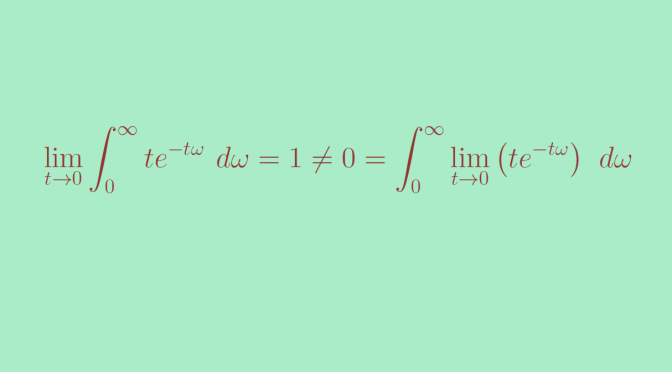Continuity under the integral sign

We consider here a measure space $$(\Omega, \mathcal A, \mu)$$ and $$T \subset \mathbb R$$ a topological subspace. For a map $$f : T \times \Omega \to \mathbb R$$ such that for all $$t \in T$$ the map $\begin{array}{l|rcl} f(t, \cdot) : & \Omega & \longrightarrow & \mathbb R \\ & \omega & \longmapsto & f(t,\omega) \end{array}$ is integrable, one can define the function $\begin{array}{l|rcl} F : & T & \longrightarrow & \mathbb R \\ & t & \longmapsto & \int_\Omega f(t,\omega) \ d\mu(\omega) \end{array}$

Following theorem is well known (and can be proven using dominated convergence theorem):

THEOREM for an adherent point $$x \in T$$, if

• $$\forall \omega \in \Omega \lim\limits_{t \to x} f(t,\omega) = \varphi(\omega)$$
• There exists a map $$g : \Omega \to \mathbb R$$ such that $$\forall t \in T, \, \forall \omega \in \Omega, \ \vert f(t,\omega) \vert \le g(\omega)$$

then $$\varphi$$ is integrable and $\lim\limits_{t \to x} F(t) = \int_\Omega \varphi(\omega) \ d\mu(\omega)$
In other words, one can switch $$\lim$$ and $$\int$$ signs.

We provide here a counterexample showing that the conclusion of the theorem might not hold if $$f$$ is not bounded by a function $$g$$ as supposed in the premises of the theorem.

Let’s consider $\begin{array}{l|rcl} f : & [0, \infty) \times [0, \infty) & \longrightarrow & \mathbb R \\ & (t, \omega) & \longmapsto & t e^{-t \omega} \end{array}$ For all $$t \in [0,\infty)$$, the map $$\omega \mapsto f(t,\omega)$$ is integrable on $$[0, \infty)$$. Therefore, one can define $\begin{array}{l|rcl} F : & [0, \infty) & \longrightarrow & \mathbb R \\ & t & \longmapsto & \int_0^\infty t e^{-t \omega} \ d\omega\end{array}$

Moreover for all $$\omega \in [0,\infty)$$, $$f(0,\omega)=0$$ hence $$F(0)=0$$. And for $$t \neq 0$$, $F(t)=\int_0^\infty t e^{-t \omega} \ d\omega = 1.$

Finally $\lim\limits_{t \to 0} \int_0^\infty t e^{-t \omega} \ d\omega = 1 \neq 0 = \int_0^\infty \lim\limits_{t \to 0} \left( t e^{-t \omega} \right) \ d\omega$

One can verify that for $$\omega \in (0,\infty)$$, the maximum of $$t \mapsto t e^{-t \omega}$$ is obtained for $$t = \frac{1}{\omega}$$ and is equal to $$\frac{1}{e \omega}$$. Hence a map $$g$$ as defined in the hypotheses of the theorem is forced to satisfy the inequality $$\frac{1}{e \omega} \le g(\omega)$$ and cannot be integrable.